## Algorithm Competition Finals SummaryThursday, May 15, 2008
Introduction by d000hg

Discuss this competition

### tomek is the new Algorithm Champion!The Finals play-by-play can be found here.

#### CoolFunction

Let's call the function f(x) = |x - a| a primitive with center at a. In this problem, we must express the given function as a sum of primitives. Any such expression will be called a solution.

First, let's find how many primitives with centers at 1, 2, ..., n-2 we should use. Let a, 1 ≤ a ≤ n - 2 be the some fixed center. Choose an arbitrary solution and let's left be the number of primitives in this solution with centers smaller than a, at be the number of primitives with centers at a and right be the number of primitives with centers larger than a. Consider the change of function f when going from point a-1 to a: left primitives increase the function by 1, and at+right primitives decrease it by 1. Similarly, when we go from point a to point a+1, left+at primitives give increment by 1, and right primitives give decrement. So we have the following 2 equations:

```    f(a) - f(a-1) = left - at - right
f(a+1) - f(a) = left + at - right
```

Subtract the second equation from the first one and divide by 2 to get:

```    at = (f(a+1) - f(a) - f(a) + f(a-1)) / 2
```

Using this equation we can uniquely determine the number of primitives with centers at 1..n-2. Now let's check the primitives with other centers. Denote the number of primitives with centers at a ≤ 0 as left, the number of primitives with centers at 1 ≤ a ≤ n-2 as middle (we already know the value of middle) and the number of primitives with centers at a ≥ n-1 as right. Acting in similar way as we did previously it's possible to write the following equations for f(1) - f(0) and f(n-1) - f(n-2):

```    f(1) - f(0) = left - middle - right
f(n-1) - f(n-2) = left + middle - right
```

Sum the equations and divide by 2 to get the value of left - right:

```    left - right = (f(1) - f(0) + f(n-1) - f(n-2)) / 2
```

If left < right, let's call the range a ≤ 0 minor and the range a ≥ n-1 major. If left > right, then the range a ≥ n-1 is minor and the range a ≤ 0 is major. If left = right, then both ranges can be referred to as minor or major. Let's prove the following property: at most one primitive with center at any minor range can be present in the optimal solution. The idea is to show that any solution containing two or more such primitives can be improved. Note that if solution contains two primitives with centers at la ≤ 0 and ra ≥ n-1, then these two primitives increase each of values f(0), f(1), ..., f(n-1) by |la| + |ra|. Suppose we have some solution with two or more primitives on the minor side. Then it must have at least two primites on the major side, too. It means, that there are 4 primitives with centers at la1, la2 ≤ 0 and ra1, ra2 ≥ n-1. But the same effect can be achieved by replacing these 4 primitives by just 2 with centers at la1+la2 and ra1+ra2. This is an improvement, as our new solution contains less primitives than the previous one.

Now let's prove one more property. Suppose left > right. We want to prove that the optimal solution contains at least left - right - 1 primitives with centers at 0. Alternatively, it can be formulated as "the optimal solution contains at most one primitive with center at a < 0". The proof is similar to the previous one. If we have two primitives with centers at la1 < 0 and la2 < 0, then we can replace them with by primitives with centers at 0 and la1+la2. If we choose la1 and la2 as the smallest centers of primitives among all centers ≤ 0, our replacement will be an improvement, because it will give lexicographically smaller result. Similar argumentation shows that if left < right, then we have at least right - left - 1 primitives with centers at n-1.

We are ready to finish the solution. If leftright, add |right - left| - 1 primitives with centers at 0 or n-1 (depending on which range is major). Now we need to add at most one primitive to each side (adding more primitives would lead to having more than one primitive in minor range and thus would not lead to optimal solution). As there are at most 51 positions to try at each side, the conceptually simplest way to determine which primitives to add is brute force. If we find more than one solution, tiebreaker will be used to choose the optimal one among them.

by misof

#### CraneSort

Consider an input with N crates. We have to answer three questions: First, where (relative to the current leftmost crate) will the final configuration of crates start? Second, in which order will the crate types A, B, C, and D be arranged? And third, once we answer the first two questions and thus determine the final configuration, how can we compute the minimal number of moves to reach it?

For the first question, it is enough to realize that there are just 2N-1 possible "offsets" such that the initial and the final configuration intersect. All other offsets give essentially the same situation where the initial and the final configuration don't intersect (and thus we need exactly N moves). The simplest way how to answer the first question is simply to try all offsets from -N to N-1.

The same is true for the second question. There are only 24 permutations of crate types. We can try them all out, and pick the best one.

This leaves us with the most interesting part: Having a fixed final configuration, what is the minimum number of moves we need to produce it?

This will best be explained by a series of examples.

```example:  1    2     3      4    5     6      7      8
initial:  A-   AB-   ABB-   CD   CDA   CDCD   CCDD   ABCD
final:  -A   -AB   -ABB   DC   DAC   DCDC   DDCC   BADC
```
1. This case is simple: one move is needed to move the crate.
2. We have to move crate A to a place currently occupied by crate B, and crate B to a place that is currently free. When done in proper order, this can be done in two moves, and obviously can't be done in less than two.
3. Same as example 2. We can ignore crates that stay on the same place, in the optimal solution we won't move them.
4. In this case, none of the crates can be placed on the desired place in the first move. Thus we need at least three moves. Obviously, it is doable in three moves.
5. The same with a larger cycle. In the first move put one crate into a free spot, sequentially put other crates in place, and finally return the one crate back.
6. Here we have twice the case from example 4. The important observation is that we can solve this configuration in 5 moves – removing one crate is enough to be able to move all the others into correct places.
7. This is the same as example 6, both can be described as "2x(C to D), 2x(D to C)".
8. This case differs from example 6. Here we have two disjoint groups, one involving crate types A and B, and the other involving crate types C and D. We have to solve each group separately, resulting in 6 moves.

We can now generalize these observations into an algorithm. We can represent the situation as a directed graph. The vertices will be -, A, B, C, and D. For each place, we will add a directed edge from the initial crate type to the final crate type in that place. Each edge starting in A, B, C, or D represents a crate. Loops are edges that don't need to be moved, the other edges represent the required moves.

Connected components in this graph represent groups of crate types we have to handle separately. If a component does not contain the vertex -, we need to make one extra move to create a free space. Thus the total number of moves for these crate types is one plus the number of non-loop edges in this component. For the component containing the vertex - the number of moves is the number of non-loop edges not originating in the vertex -.

by legakis

#### SnakesOnAPlane

I will first describe a brute-force solution to this problem that only works for small inputs, and then show how it can be optimized with memoization.

It helps to simplify the problem in two ways. First, rather than saying snakes can begin and end on the border of the rectangle, let's assume that snakes can extend off the edges. Second, rather than saying snakes can have adjacent endpoints in the interior of the rectangle, let's assume that snakes can actually form a closed loop with no endpoints at all. With these changes, a solution that looks like this:we could now think of as looking like this:Note that this does not change the problem in any way, it's just a different way to think about it. The benefit is that now there are only 6 possible shapes of snakes to fill every non-barrier square: 4 snake segments with 90-degree bends and 2 straight snake segments.

A simple-brute force recursive solution to this problem would be to fill in the empty squares one at a time, left-to-right then top-to-bottom, trying each shape of snake segment that is compatible with the segments already placed in adjacent squares. There are several constraints that limit the shapes that can go in a square, depending on whether the segment to the left and the segment above extend into the square that you are processing. For example, if neither of them do, then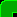is the only segment that can fit. If both of them do, then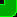is the only one. If a snake extends from above but not to the left, then only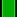and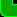segments can fit. Similarly, if a snake extends from the left but not above, then only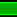and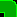segments can.

The recursive function can return the minimum number of snakes necessary to add from this point forward in order to complete the rectangle. Adding asegment creates a new snake, and increases the count by one. Adding asegment joins two snakes, and decreases the count by one. Note than any snake that forms a loop will contain an equal number ofandsegments, so the net effect on the count will be zero. Adding aorsegment in the top row or adding aorsegment in the left column also creates a new snake, increasing the count by 1. Adding any of these 4 segments anywhere else simply extends an existing snake, and does not affect the count.

Memoization can be used to speed up this algorithm for larger inputs. The key is that to process any given square, all you need to keep track of is which of the previously processed squares have snakes that extend into unprocessed squares. With an input of size WxH, there are at most W unprocessed squares with a processed square above and at most 1 unprocessed square with a processed square to the left, so this information can be encoded in (W+1) bits. The number of possible states is therefore W * H * 2W+1, which is only 1,179,648 for the maximum input size of 12x12.